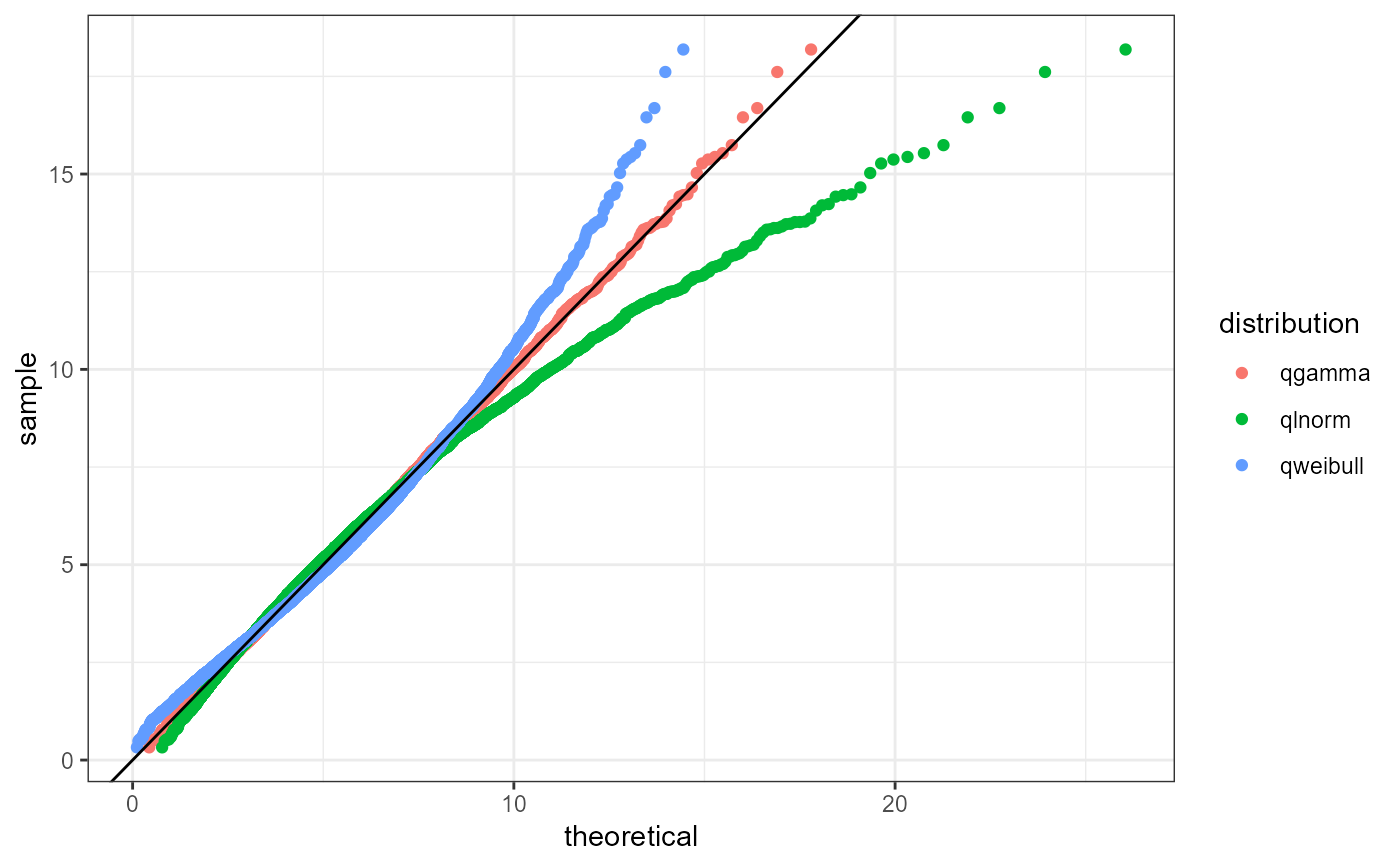Q-Q Plot

plot_qq(x, fits)

## Arguments

x numeric vector of sample data a list object produced from fit_univariate, fit_empirical, or fit_univariate_man

## Value

ggplot of quantile-quantile comparison of theoretical distribution

## Examples

library(ggplot2)
set.seed(37)
x <- rgamma(10000, 5)
dists <- c('gamma', 'lnorm', 'weibull')
fits <- lapply(dists, fit_univariate, x = x)
plot_qq(x, fits) +
theme_bw()